PSABM6WN - Student Facing Task---Acc7.5 Lesson 17 Organizing Data (8.SP.A.1, 8.SP.A)

Here is a table of data. Each row shows two measurements of a triangle.

length of short side (cm) length of perimeter (cm)
0.25 1
2 7.5
6.5 22
3 9.5
0.5 2
1.25 3.5
3.5 12.5
1.5 5
4 14
1 2.5

What do you notice? What do you wonder?

Part A)

Here is the table of isosceles right triangle measurements from the warm-up and an empty table.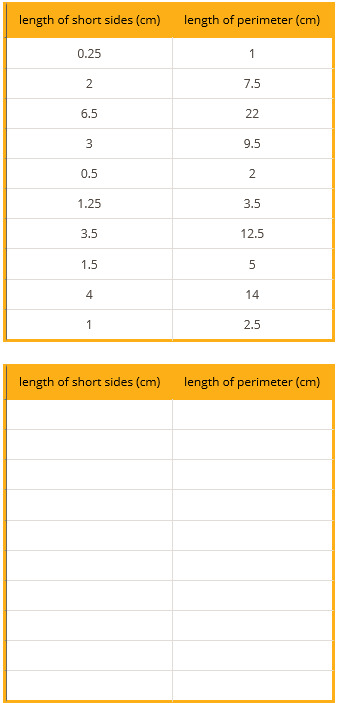How can you organize the measurements from the first table so that any patterns are easier to see? Write the organized measurements in the empty table.

Create and fill in the table using the table button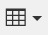Part B)

For each of the following lengths, estimate the perimeter of an isosceles right triangle whose short sides have that length. Explain your reasoning for each triangle.

Perimeter if length of short sides is 0.75 cm:

Part C)

Part D)

Perimeter if lengths of short sides is 5 cm:

Part E)

Part F)

Perimeter if the length of short sides is 10 cm:

Part G)

Part A)

In addition to the graphic representations of data you have learned, there are others that make sense in other situations. Examine the maps showing the results of the elections for United States president for 2012 and 2016. In red are the states where a majority of electorate votes were cast for the Republican nominee. In blue are the states where a majority of the electorate votes were cast for the Democrat nominee.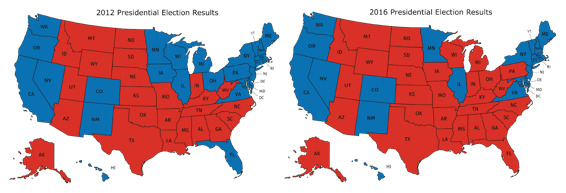What information can you see in these maps that would be more difficult to see in a bar graph showing the number of electorate votes for the 2 main candidates?

Part B)

Why are these representations appropriate for the data that is shown?

Part A)

Here are four scatter plots. Your teacher will give you four tables of data.

• Match each table with one of the scatter plots.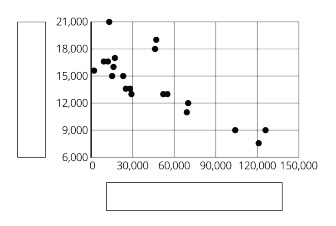Which table matches with this graph?

Select one:
Part B)

Use information from the tables to label the axes for each scatter plot.

Label for x-axis:

Label for y-axis:

Part C)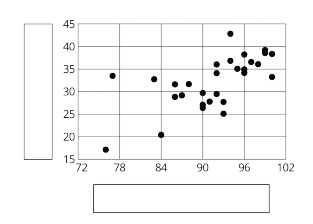Which table matches with this graph?

Select one:
Part D)

Use information from the tables to label the axes for each scatter plot.

Label for x-axis:

Label for y-axis:

Part E)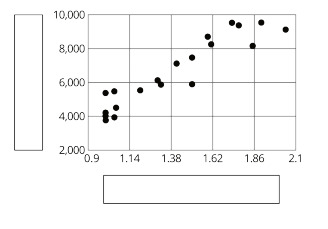Which table matches with this graph?

Select one:
Part F)

Use information from the tables to label the axes for each scatter plot.

Label for x-axis:

Label for y-axis:

Part G)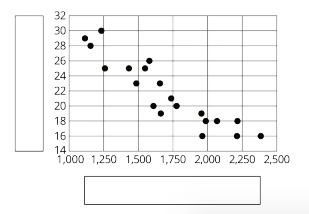Which table matches with this graph?

Select one:
Part H)

Use information from the tables to label the axes for each scatter plot.

Label for x-axis:

Label for y-axis: